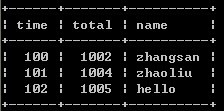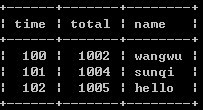### SQL 结构化查询语言

SQL中经常遇到如下情况，在一张表中有两条记录基本完全一样，某个或某几个字段有些许差别，1、select time,max(total) as total,name from timeand group by time;//取记录中total最大的值2、select * from timeand as a where not exists(select 1 from timeand where a.time = time and a.total<total);select * from timeand as a where not exists(select 1 from timeand where a.time = time and a.total<total);

select * from timeand as a where not exists(select 1 from timeand where a.time = time and (a.total<total or (a.total=total and a.outtotal<outtotal)));

SQL中遇到多条相同内容只取一条的最简单实现方法

SQL中EXISTS函数的用法

SQL中 decode()函数简介

SQL中print、sp_helptext的限制与扩展

SQL中Merge用法详解
SQL中distinct的用法（四种示例分析）